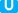## 物品栏分区

### 垃圾箱（1 栏位）

1. 译注：游戏官方中文版中将此位置的名称“Trash”（名词）译为“丢弃”，此为错译，本 wiki 中对此将统一使用“垃圾箱”。但“Trash slot”依然使用“丢弃栏”，“Trash”（动词）也依然使用“丢弃”。

### 装备（5 栏位）

\l!--\n --\g\{\{@@@@\_version\!\{\{#var:_s_mode\}\}\!nl\e\{\{#var:_s_nl\}\}\!small\e\{\{#var:_s_small\}\}\}\}\l!--\_\$short\_has\_no\_effect\_in\_icon\_mode\n --\g \l!--\n --\g\{\{@@@@\_version\!\{\{#var:_s_mode\}\}\!nl\e\{\{#var:_s_nl\}\}\!small\e\{\{#var:_s_small\}\}\}\}\l!--\_\$short\_has\_no\_effect\_in\_icon\_mode\n --\g \l!--\n --\g\{\{@@@@\_version\!\{\{#var:_s_mode\}\}\!nl\e\{\{#var:_s_nl\}\}\!small\e\{\{#var:_s_small\}\}\}\}\l!--\_\$short\_has\_no\_effect\_in\_icon\_mode\n --\g \l!--\n --\g\{\{@@@@\_version\!\{\{#var:_s_mode\}\}\!nl\e\{\{#var:_s_nl\}\}\!small\e\{\{#var:_s_small\}\}\}\}\l!--\_\$short\_has\_no\_effect\_in\_icon\_mode\n --\g \l!--\n --\g\{\{@@@@\_version\!\{\{#var:_s_mode\}\}\!nl\e\{\{#var:_s_nl\}\}\!small\e\{\{#var:_s_small\}\}\}\}\l!--\_\$short\_has\_no\_effect\_in\_icon\_mode\n --\g
///////独有内容：此章节的信息适用于的泰拉瑞亚

### 装备染料（5 栏位）

\l!--\n --\g\{\{@@@@\_version\!\{\{#var:_s_mode\}\}\!nl\e\{\{#var:_s_nl\}\}\!small\e\{\{#var:_s_small\}\}\}\}\l!--\_\$short\_has\_no\_effect\_in\_icon\_mode\n --\g \l!--\n --\g\{\{@@@@\_version\!\{\{#var:_s_mode\}\}\!nl\e\{\{#var:_s_nl\}\}\!small\e\{\{#var:_s_small\}\}\}\}\l!--\_\$short\_has\_no\_effect\_in\_icon\_mode\n --\g \l!--\n --\g\{\{@@@@\_version\!\{\{#var:_s_mode\}\}\!nl\e\{\{#var:_s_nl\}\}\!small\e\{\{#var:_s_small\}\}\}\}\l!--\_\$short\_has\_no\_effect\_in\_icon\_mode\n --\g \l!--\n --\g\{\{@@@@\_version\!\{\{#var:_s_mode\}\}\!nl\e\{\{#var:_s_nl\}\}\!small\e\{\{#var:_s_small\}\}\}\}\l!--\_\$short\_has\_no\_effect\_in\_icon\_mode\n --\g \l!--\n --\g\{\{@@@@\_version\!\{\{#var:_s_mode\}\}\!nl\e\{\{#var:_s_nl\}\}\!small\e\{\{#var:_s_small\}\}\}\}\l!--\_\$short\_has\_no\_effect\_in\_icon\_mode\n --\g
///////独有内容：此章节的信息适用于的泰拉瑞亚

## 物品栏子菜单

### 相机模式

\l!--\n --\g\{\{@@@@\_version\!\{\{#var:_s_mode\}\}\!nl\e\{\{#var:_s_nl\}\}\!small\e\{\{#var:_s_small\}\}\}\}\l!--\_\$short\_has\_no\_effect\_in\_icon\_mode\n --\g \l!--\n --\g\{\{@@@@\_version\!\{\{#var:_s_mode\}\}\!nl\e\{\{#var:_s_nl\}\}\!small\e\{\{#var:_s_small\}\}\}\}\l!--\_\$short\_has\_no\_effect\_in\_icon\_mode\n --\g
///////独有内容：此章节的信息适用于的泰拉瑞亚

## 备注

• 如果所有热键栏和收纳栏都已被占据，则不会捡起新物品，除非玩家携带有。
• 只有出现在主物品栏中的物品才可用于制作。不过，在\{\{comma/item\!\l!--\n --\g\{\{#var:_s_bi_start\}\}\l!--\_bold/italic\_opening\_tags\n --\g\{\{@@@@\_version\!\{\{#var:_s_mode\}\}\!short\e\{\{#var:_s_short\}\}\!nl\e\{\{#var:_s_nl\}\}\!small\e\{\{#var:_s_small\}\}\}\}\l!--\n --\g\{\{#var:_s_bi_end\}\}\l!--\_bold/italic\_closing\_tags\n --\g\}\}\{\{comma/item\!\l!--\n --\g\{\{#var:_s_bi_start\}\}\l!--\_bold/italic\_opening\_tags\n --\g\{\{@@@@\_version\!\{\{#var:_s_mode\}\}\!short\e\{\{#var:_s_short\}\}\!nl\e\{\{#var:_s_nl\}\}\!small\e\{\{#var:_s_small\}\}\}\}\l!--\n --\g\{\{#var:_s_bi_end\}\}\l!--\_bold/italic\_closing\_tags\n --\g\}\}中，也可以使用当前已经打开的收纳物品中的物品来制作。
• 在物品栏中的物件将带着转移到其他的服务器和世界。这也包括已装备的盔甲、钱币栏中的钱币、和弹药栏中的弹药。
•Wii U 中，如果点击关闭按钮，玩家的人物会跳起。

## 小贴士

• 丢弃栏提供了一个额外的物品栏栏。可以将一件物品临时放在其中以腾出主物品栏空间。但要小心，因为如果将任何其他物品放入丢弃栏、死亡、或退出游戏，都会删除该栏位中的东西。
• 另一个增加物品栏栏位的方法是一直将猪猪存钱罐保险箱、及放在主物品栏中随身携带，因为这三个能同时带上放在其中的物品。要注意猪猪存钱罐要求有一个平坦表面物品才能放下，比如工作台桌子、或平台。而并无此限制。
• 将多余的物品卖给 NPC 而非直接丢掉是个赚钱的好办法。

## 历史

• 电脑版 1.3.3.2
• 游戏摇杆的按键绑定菜单中现在有一个滑块，可以调整物品栏导航速度。
• 默认的游戏摇杆菜单/物品栏菜单的速度也调快了，另外也可以通过更快速地点击来加快速度。
• 电脑版 1.3.0.1
• 改造了人物选择菜单来显示额外的人物信息。
• 你现在可以通过按 F1 来切换屏幕截图模式。这使得可以在游戏中捕捉更大的屏幕截图。
• 添加了矿车栏、坐骑栏、宠物栏和抓钩栏。
• 添加了按 Alt + 左键点击来收藏物品的能力。被收藏的物品不受快速丢弃、快速堆叠、或存放功能的影响。
• 增加了一个物品栏按钮来快速堆叠至附近的宝箱上，让库存管理更加快捷。
• 快速堆叠现在会在你的宝箱中为你物品栏中所拥有的物品创建新的堆叠（如果适用）。
• 装备栏现在会显示栏位目的相应的图片。
• 在丢弃栏中的物品现在会在人物死亡时删除。
• 电脑版 1.2.4.1：修正了当你右键点击物品栏中的物品时的制作漏洞。
• 电脑版 1.2.4：修正了异常的物品堆叠大小会导致游戏在保存时崩溃的 Bug。修正了可以通过购买与出售可堆叠物品获利的漏洞。
• 电脑版 1.2
• 收纳物品栏栏位数提升至 40（之前是 30）。
• 引入了染料栏。
• 很多物品的最大堆叠数由 250 提升到999。
• 电脑版 1.1
• 房屋菜单添加到主物品栏中了。
• 现在，如果关闭自动暂停，位于主物品栏中的物品可以正常使用而无需将其放置到热键栏中。
• 电脑版 1.0.6
• 弹药栏和丢弃栏添加到主物品栏中了。
• 物品栏栏位会根据正在查看哪个物品栏来给予独特的颜色。
• 热键栏栏位现在会显示相应的快捷键。
• 放着远程武器的热键栏栏位现在在上面会正确显示所用弹药的数量了。
• 热键栏现在可以通过点击左边的挂锁来锁定。
• 右键点击物品栏中的配饰或盔甲会自动装备它。
• 不再能够通过物品栏各方框之间的空白位置来丢下物品了。
• 新得到的物品现在会从物品栏的右下角开始放置而非左上角。
• 电脑版 1.0.5
• 热键栏现在会显示所选定物品的名称。
• 现在在物品栏中会显示总防御力。
• 电脑版 1.0.4：添加了一个新的选项，在单人游戏中当物品栏处于打开状态时会暂停游戏，称为自动暂停。此选项默认关闭。